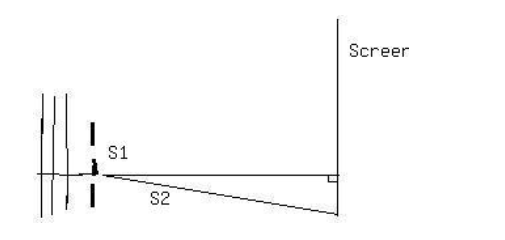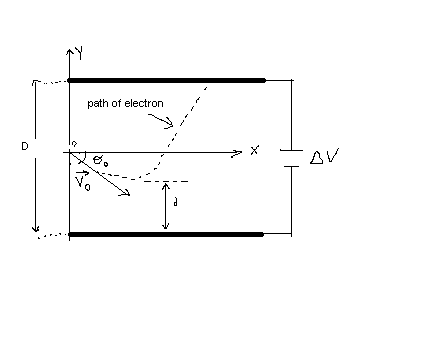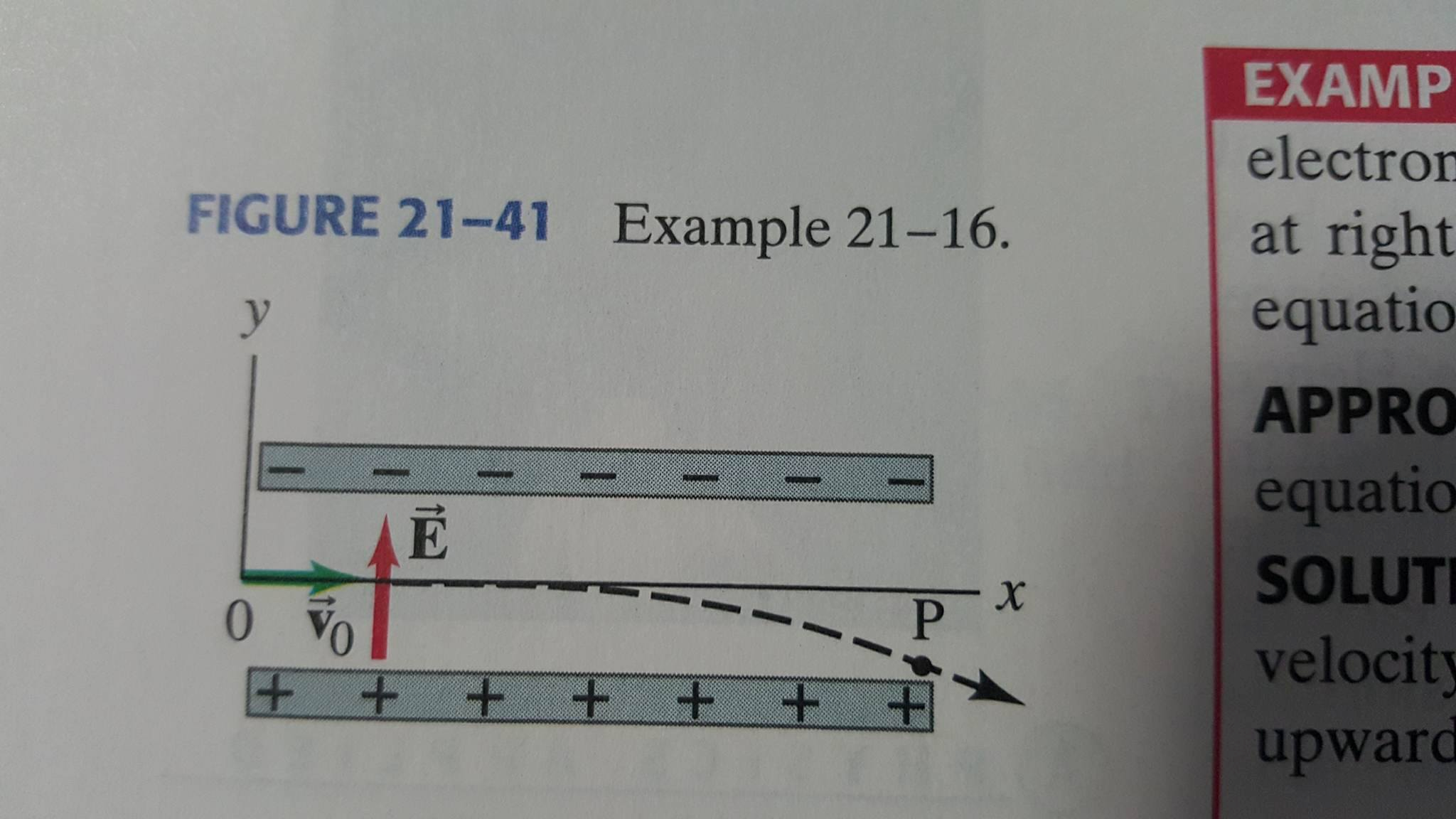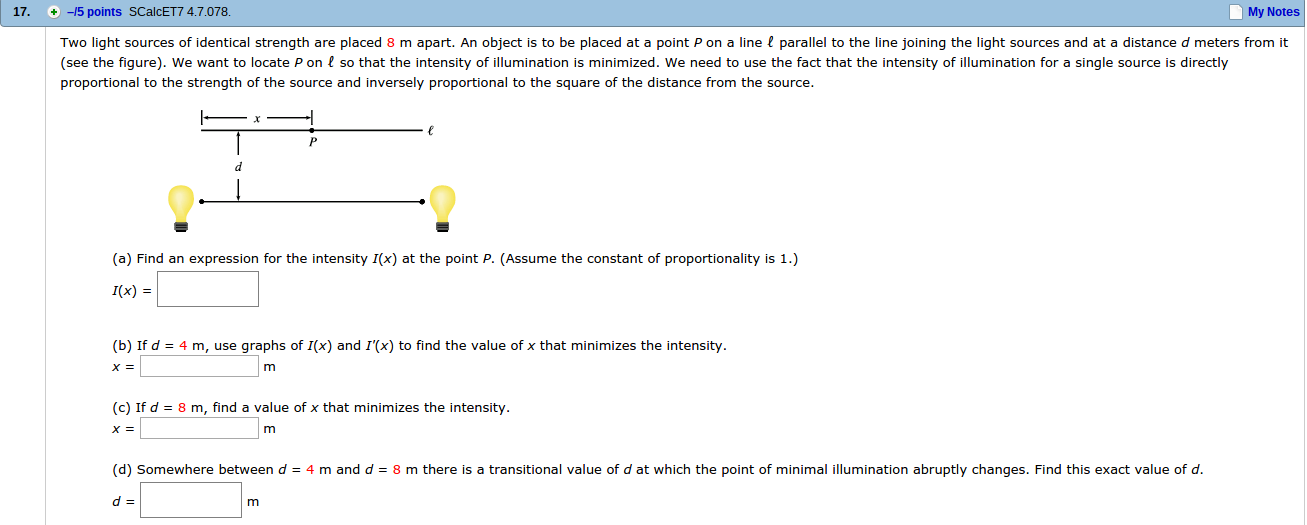Ask question

# 1. S1 and S2, shown above, are thin parallel slits in an opaqueplate. A plane wave of wavelength λ is incident from the leftmoving in a direction perpendicular to the plate. On a screenfar from the slits there are maximums and minimums in intensity atvarious angles measured from the center line. As the angle isincreased from zero, the first minimum occurs at 3 degrees. Thenext minimum occurs at an angle of- A. 4.5 degrees B. 6 degrees C. 7.5 degrees D. 9 degrees E. 12 degrees# 1. S1 and S2, shown above, are thin parallel slits in an opaqueplate. A plane wave of wavelength λ is incident from the leftmoving in a direction perpendicular to the plate. On a screenfar from the slits there are maximums and minimums in intensity atvarious angles measured from the center line. As the angle isincreased from zero, the first minimum occurs at 3 degrees. Thenext minimum occurs at an angle of- A. 4.5 degrees B. 6 degrees C. 7.5 degrees D. 9 degrees E. 12 degrees

Question
Otherasked 2021-05-181. S1 and S2, shown above, are thin parallel slits in an opaqueplate. A plane wave of wavelength λ is incident from the leftmoving in a direction perpendicular to the plate. On a screenfar from the slits there are maximums and minimums in intensity atvarious angles measured from the center line. As the angle isincreased from zero, the first minimum occurs at 3 degrees. Thenext minimum occurs at an angle of-
A. 4.5 degrees
B. 6 degrees
C. 7.5 degrees
D. 9 degrees
E. 12 degrees

## Answers (1)2021-05-20
6 degrees
$$\displaystyle{d}{\sin{{0}}}={m}\lambda$$
$$\displaystyle{{\sin{{3}}}^{{\circ}}=}{\left({1}\right)}{\left(\frac{\lambda}{{d}}\right)}$$
$$\displaystyle{\left(\frac{\lambda}{{d}}\right)}={.052}$$
When m=2,
$$\displaystyle{\sin{{0}}}={.105}$$ therefore theta is 6 degrees.

### Relevant Questionsasked 2021-05-12
An electron is fired at a speed of $$\displaystyle{v}_{{0}}={5.6}\times{10}^{{6}}$$ m/s and at an angle of $$\displaystyle\theta_{{0}}=–{45}^{\circ}$$ between two parallel conductingplates that are D=2.0 mm apart, as in Figure. Ifthe potential difference between the plates is $$\displaystyle\triangle{V}={100}\ {V}$$, determine (a) how close d the electron will get to the bottom plate and (b) where the electron will strike the top plate.asked 2021-05-02
Suppose electrons enter the uniform electric field midwaybetween two plates, moving at an upward 45 degree angle as shown.What maximum speed can the electrons have if they are to avoidstriking the upper plate. Ignore fringing of the field. Upper plate is negatively charged bottom plate is positivelycharged length of plate is 6cm and distance from top of top plateto bottom of bottom plate is 1cm.
$$\displaystyle{E}={5.0}\times{10}^{{3}}\frac{{N}}{{C}}$$asked 2021-04-22
Nerve cells are long, thin cylinders along which electric disturbances (nerve impulses) travel. The cell membrane if a typical nerve cell consists of an inner and outer wall separated by a distance of $$\displaystyle{0.10}\mu{m}$$. The electric field within the cell membrane is $$\displaystyle{7.0}\cdot{10}^{{{5}}}$$ N/C. Approximating the cell membrane as a parallel plate capacitor, determine the magnitude of the charge density on the inner and outer cell walls.asked 2021-05-16
Look Out! A snowball rolls off a barn roof that slopes downward at an angle of 40 degrees . The edge of the roof is 14.0 m above the ground, and the snowball has a speed of 7.00 m/s as it rolls off the roof. Ignore air resistance.
A man 1.9 m tall is standing 4.0 m from the edge of the barn. Will he be hit by the snowball?asked 2021-03-12
A 75.0-kg man steps off a platform 3.10 m above the ground. Hekeeps his legs straight as he falls, but at the moment his feettouch the ground his knees begin to bend, and, treated as aparticle, he moves an additional 0.60 m before coming torest.
a) what is the speed at the instant his feet touch theground?
b) treating him as a particle, what is his acceleration(magnitude and direction) as he slows down, if the acceleration isassumed to be constant?
c) draw his free-body diagram (see section 4.6). in termsof forces on the diagram, what is the net force on him? usenewton's laws and the results of part (b) to calculate the averageforce his feet exert on the ground while he slows down. expressthis force in newtons and also as a multiple of his weight.asked 2021-05-05
A golf ball lies 2.00 m directly south of the hole on a levelgreen. On the first putt, the ball travels 3.00 m along astraight-line path at an angle of 5 degrees east of north; on thesecond putt, it travels a straight-line distance of 1.20 m at anangle of 6 degrees south of west.
What would be the displacement ofa third putt that would put the ball in the hole?
The answer in the back of the book is 1.3 m at 43 degreessouth of east. How do I get that?asked 2021-04-03
At what angle will the electrons leave the uniform electric field at the end of the parallel plates? Assume the plates are 4.9 cm long, $$\displaystyle{E}={5.0}\times{10}^{{3}}$$ and $$\displaystyle{v}_{{0}}={1.00}\times{10}^{{7}}$$ m/s. Ignore fringing on the field.asked 2021-02-10
Two light sources of identical strength are placed 8 m apart. An object is to be placed at a point P on a line ? parallel to the line joining the light sources and at a distance d meters from it (see the figure). We want to locate P on ? so that the intensity of illumination is minimized. We need to use the fact that the intensity of illumination for a single source is directly proportional to the strength of the source and inversely proportional to the square of the distance from the source.asked 2021-05-12
4.7 A multiprocessor with eight processors has 20attached tape drives. There is a large number of jobs submitted tothe system that each require a maximum of four tape drives tocomplete execution. Assume that each job starts running with onlythree tape drives for a long period before requiring the fourthtape drive for a short period toward the end of its operation. Alsoassume an endless supply of such jobs.
a) Assume the scheduler in the OS will not start a job unlessthere are four tape drives available. When a job is started, fourdrives are assigned immediately and are not released until the jobfinishes. What is the maximum number of jobs that can be inprogress at once? What is the maximum and minimum number of tapedrives that may be left idle as a result of this policy?
b) Suggest an alternative policy to improve tape driveutilization and at the same time avoid system deadlock. What is themaximum number of jobs that can be in progress at once? What arethe bounds on the number of idling tape drives?asked 2021-05-10
Hypothetical potential energy curve for aparticle of mass m
If the particle is released from rest at position r0, its speed atposition 2r0, is most nearly
a) $$\displaystyle{\left({\frac{{{8}{U}{o}}}{{{m}}}}\right)}^{{1}}{\left\lbrace/{2}\right\rbrace}$$
b) $$\displaystyle{\left({\frac{{{6}{U}{o}}}{{{m}}}}\right)}^{{\frac{{1}}{{2}}}}$$
c) $$\displaystyle{\left({\frac{{{4}{U}{o}}}{{{m}}}}\right)}^{{\frac{{1}}{{2}}}}$$
d) $$\displaystyle{\left({\frac{{{2}{U}{o}}}{{{m}}}}\right)}^{{\frac{{1}}{{2}}}}$$
e) $$\displaystyle{\left({\frac{{{U}{o}}}{{{m}}}}\right)}^{{\frac{{1}}{{2}}}}$$
if the potential energy function is given by
$$\displaystyle{U}{\left({r}\right)}={b}{r}^{{P}}-\frac{{3}}{{2}}\rbrace+{c}$$
where b and c are constants
which of the following is an edxpression of the force on theparticle?
1) $$\displaystyle{\frac{{{3}{b}}}{{{2}}}}{\left({r}^{{-\frac{{5}}{{2}}}}\right)}$$
2) $$\displaystyle{\frac{{{3}{b}}}{{{2}}}}{\left\lbrace{3}{b}\right\rbrace}{\left\lbrace{2}\right\rbrace}{\left({r}^{{-\frac{{1}}{{2}}}}\right)}$$
3) $$\displaystyle{\frac{{{3}{b}}}{{{2}}}}{\left\lbrace{3}\right\rbrace}{\left\lbrace{2}\right\rbrace}{\left({r}^{{-\frac{{1}}{{2}}}}\right)}$$
4) $$\displaystyle{2}{b}{\left({r}^{{-\frac{{1}}{{2}}}}\right)}+{c}{r}$$
5) $$\displaystyle{\frac{{{3}{b}}}{{{2}}}}{\left\lbrace{2}{b}\right\rbrace}{\left\lbrace{5}\right\rbrace}{\left({r}^{{-\frac{{5}}{{2}}}}\right)}+{c}{r}$$
...